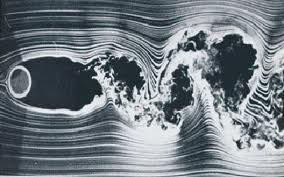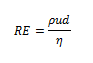History & Theory
Newton's Law of Viscosity has been used extensively in fluid dynamics to characterize the flow profile in Couette Flow. The equation derived states that the shear force between 'sheets' of fluid stacked on top of one another is equal to the velocity gradient between the sheets times the viscosity. This linear dependence of shear stress on the velocity gradient is termed Newton's Law of Viscosity.

Fluids that obey this relation are said to be Newtonian fluids - such fluids do not resist much when a force is applied. Conversely, fluids that do not obey this linear relationship will change their viscosity when a force is applied. When the viscosity of a fluid increases as a force is applied is termed shear thickening (ex. Oobleck, wet concrete). Such a fluid whose viscosity decreases as a force is applied is said to exhibit shear thinning (ex. Ketchup, blood, paint).

Laminar flow profiles in a fluid are characteristic of very 'smooth' flow lines. If one splits a block of fluid into many sheets stacked on top of one another, laminar flow occurs when there is no mixing between adjacent sheets. The sheets slide over each other and the flow properties are constant at any point within the fluid.Laminar Flow

Conversely, turbulent flow is characteristic of very chaotic flow. The sheets of fluid are moving very fast relative to one another so a mixing of adjacent sheets occurs. As a result, the flow properties fail to be constant at every point within the fluid.Turbulent Flow around a Cylinder

The Reynolds number is a dimensionless number that tells the relative importance of the inertial and viscous forces in a fluid. Fluids that have a small Reynolds exhibit laminar flow whereas fluids with very high Reynolds numbers exhibit turbulent flow.Reynolds Number Equation